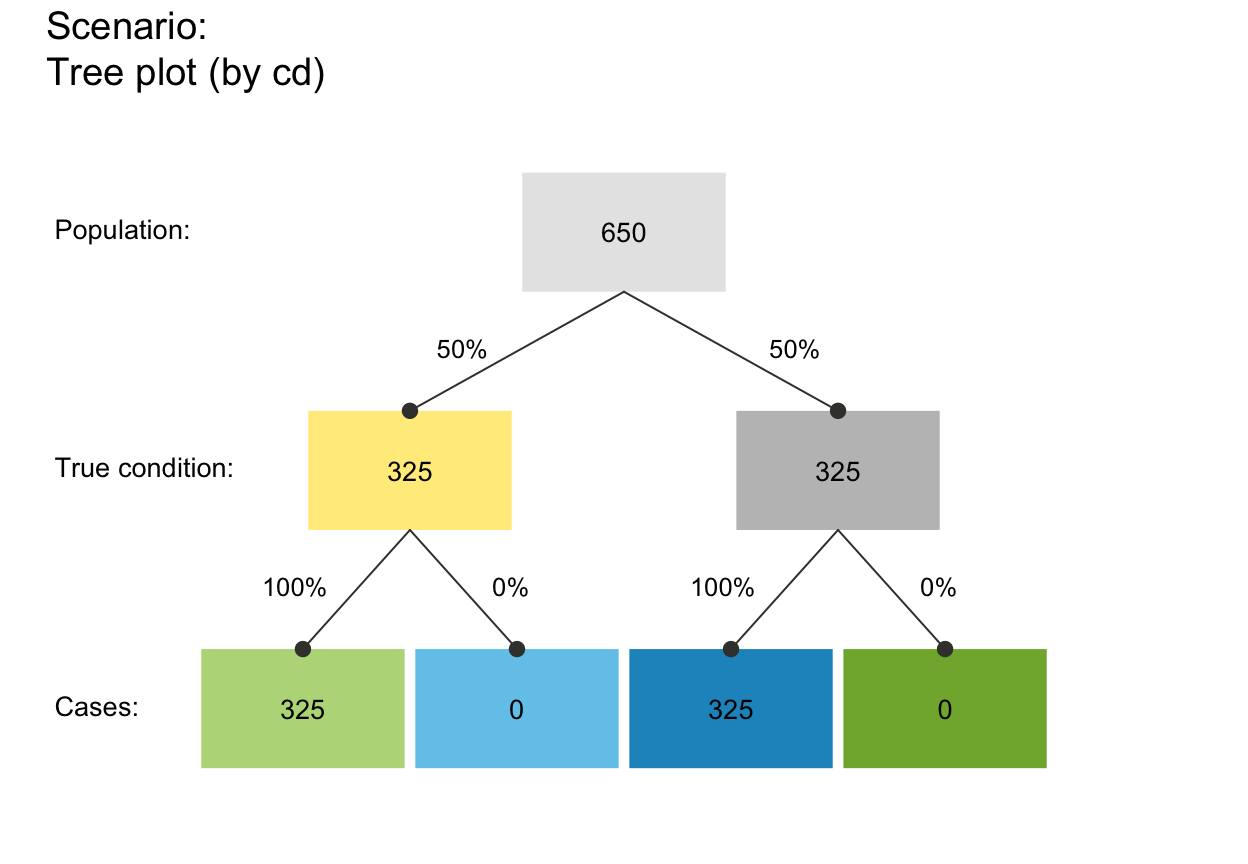is_extreme_prob_set verifies that a set of probabilities (i.e., prev, and sens or mirt, and spec or fart) describe an extreme case.

is_extreme_prob_set(prev, sens = NA, mirt = NA, spec = NA,
fart = NA)

## Arguments

prev The condition's prevalence value prev (i.e., the probability of condition being TRUE). The decision's sensitivity sens (i.e., the conditional probability of a positive decision provided that the condition is TRUE). sens is optional when is complement mirt is provided. The decision's miss rate mirt (i.e., the conditional probability of a negative decision provided that the condition is TRUE). mirt is optional when is complement sens is provided. The decision's specificity spec (i.e., the conditional probability of a negative decision provided that the condition is FALSE). spec is optional when is complement fart is provided. The decision's false alarm rate fart (i.e., the conditional probability of a positive decision provided that the condition is FALSE). fart is optional when its complement spec is provided.

## Value

A Boolean value: TRUE if an extreme case is identified; otherwise FALSE.

## Details

If TRUE, a warning message describing the nature of the extreme case is printed to allow anticipating peculiar effects (e.g., that PPV or NPV values cannot be computed or are NaN).

This function does not verify the type, range, sufficiency, or consistency of its arguments. See is_prob, is_suff_prob_set, is_complement, is_valid_prob_pair and is_valid_prob_set for these purposes.

## See also

is_valid_prob_pair verifies that a pair of probabilities can be complements; is_valid_prob_set verifies the validity of a set of probability inputs; num contains basic numeric variables; init_num initializes basic numeric variables; prob contains current probability information; comp_prob computes current probability information; freq contains current frequency information; comp_freq computes current frequency information; as_pc displays a probability as a percentage; as_pb displays a percentage as probability

Other verification functions: is_complement, is_freq, is_perc, is_prob, is_suff_prob_set, is_valid_prob_pair, is_valid_prob_set, is_valid_prob_triple

## Examples

# Identify 6 extreme cases (+ 4 variants):
is_extreme_prob_set(1, 1, NA, 1, NA)       # => TRUE + warning: N true positives#> Warning: Extreme case (prev = 1 & sens = 1):
#>   N hi (TP) cases; 0 cond_false or dec_false cases; NPV = NaN.#>  TRUEplot_tree(1, 1, NA, 1, NA, N = 100)        # => illustrates this case#> Function 'plot_tree' is deprecated; using 'plot_prism' (with by = 'cd'/'dc'/'ac') instead.#> Warning: Extreme case (prev = 1 & sens = 1):
#>   N hi (TP) cases; 0 cond_false or dec_false cases; NPV = NaN.#> Warning: Extreme case (prev = 1 & sens = 1):
#>   N hi (TP) cases; 0 cond_false or dec_false cases; NPV = NaN.#> Warning: NPV is NaN.#> Warning: FOR is NaN.#> Warning: Some derived prob values are peculiar. Check for extreme probabilities!#> Warning: accu$mcc: A denominator of 0 was corrected to 1, resulting in mcc = 0.#> Warning: Argument (prob) is no probability.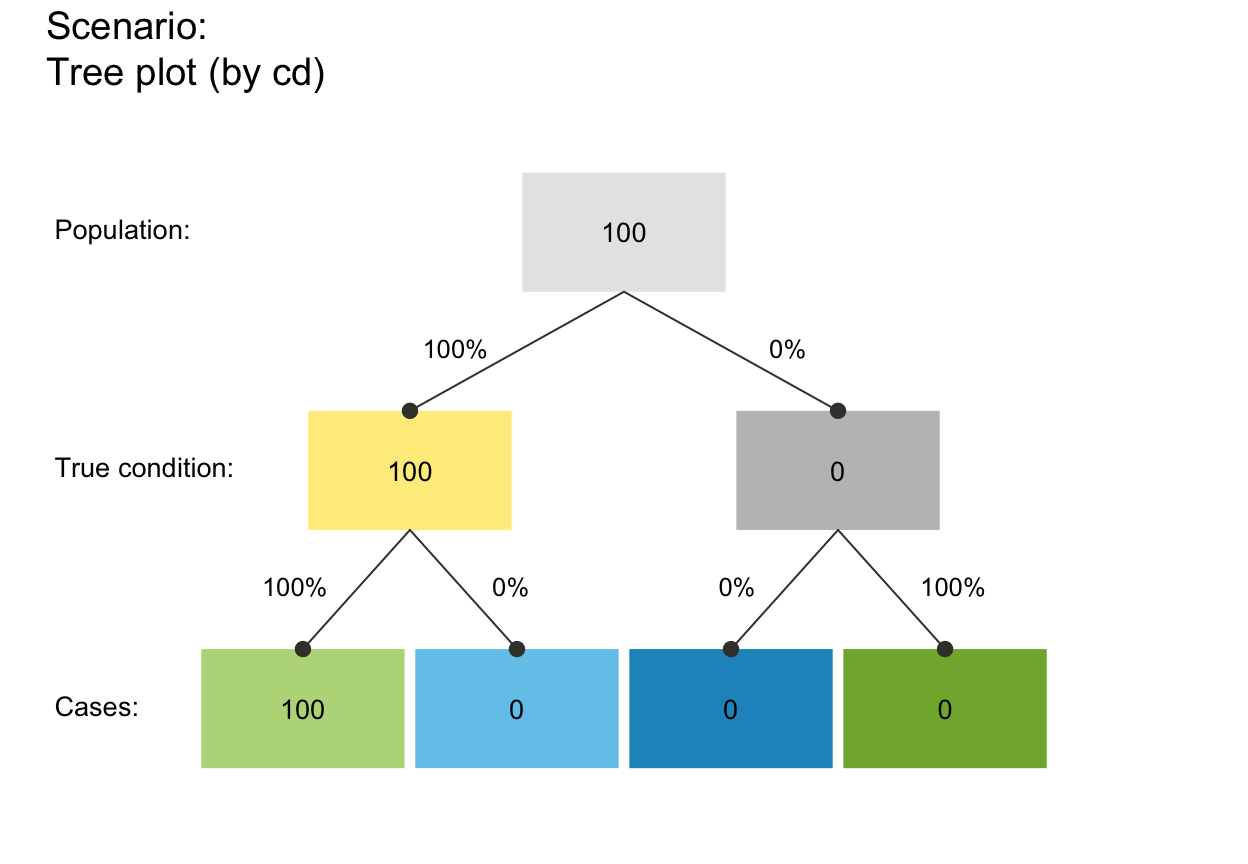is_extreme_prob_set(1, 0, NA, 1, NA) # => TRUE + warning: N false negatives#> Warning: Extreme case (prev = 1 & sens = 0): #> N mi (FN) cases; 0 cond_false or dec_true cases; PPV = NaN.#>  TRUEplot_tree(1, 0, NA, 1, NA, N = 200) # => illustrates this case#> Function 'plot_tree' is deprecated; using 'plot_prism' (with by = 'cd'/'dc'/'ac') instead.#> Warning: Extreme case (prev = 1 & sens = 0): #> N mi (FN) cases; 0 cond_false or dec_true cases; PPV = NaN.#> Warning: Extreme case (prev = 1 & sens = 0): #> N mi (FN) cases; 0 cond_false or dec_true cases; PPV = NaN.#> Warning: PPV is NaN.#> Warning: FDR is NaN.#> Warning: Some derived prob values are peculiar. Check for extreme probabilities!#> Warning: accu$mcc: A denominator of 0 was corrected to 1, resulting in mcc = 0.#> Warning: Argument (prob) is no probability.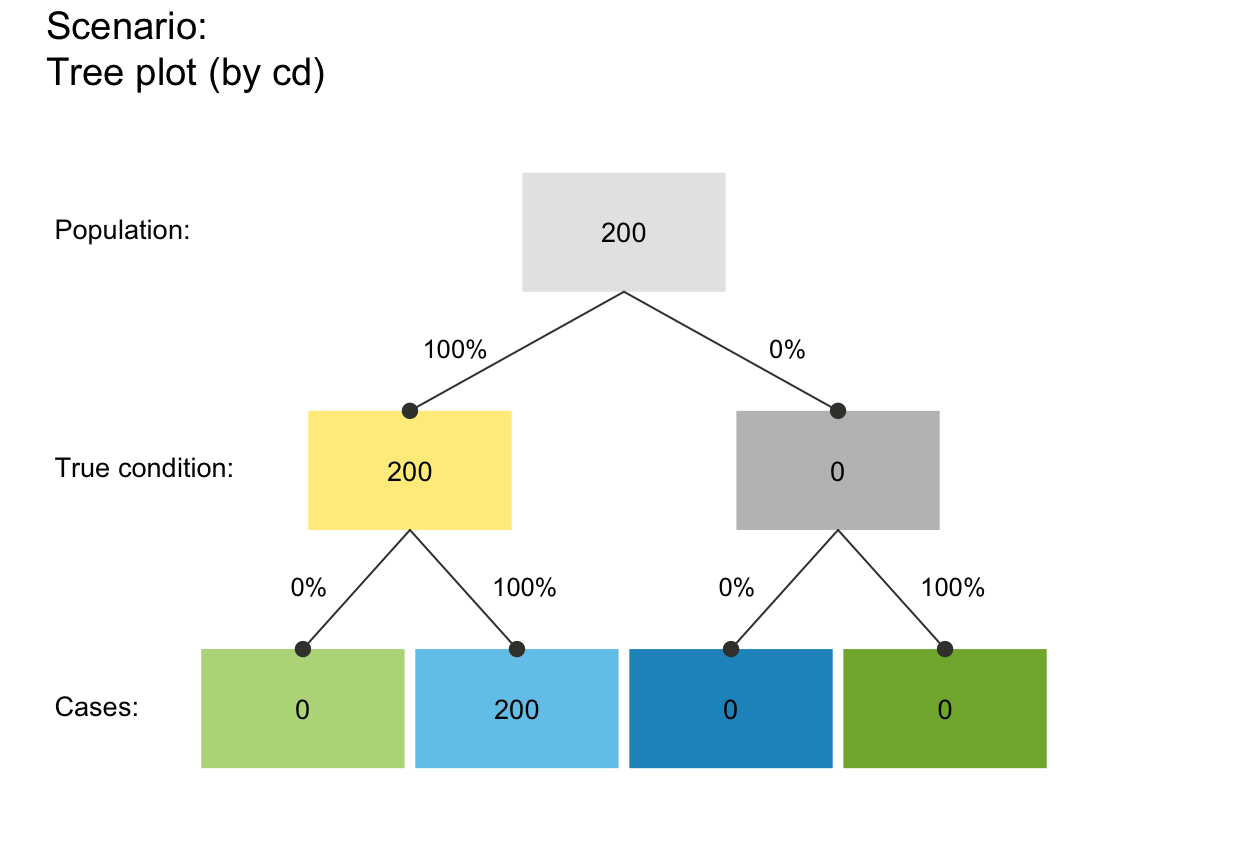sens <- .50
is_extreme_prob_set(0, sens, NA, 0, NA)    # => TRUE + warning: N false positives#> Warning: Extreme case (prev = 0 & spec = 0):
#>   N fa (FP) cases; 0 cond_true or dec_true cases; PPV = NaN.#>  TRUEplot_tree(0, sens, NA, 0, N = 300)         # => illustrates this case#> Function 'plot_tree' is deprecated; using 'plot_prism' (with by = 'cd'/'dc'/'ac') instead.#> Warning: Extreme case (prev = 0 & spec = 0):
#>   N fa (FP) cases; 0 cond_true or dec_true cases; PPV = NaN.#> Warning: Extreme case (prev = 0 & spec = 0):
#>   N fa (FP) cases; 0 cond_true or dec_true cases; PPV = NaN.#> Warning: NPV is NaN.#> Warning: FOR is NaN.#> Warning: Some derived prob values are peculiar. Check for extreme probabilities!#> Warning: accu$mcc: A denominator of 0 was corrected to 1, resulting in mcc = 0.#> Warning: Argument (prob) is no probability.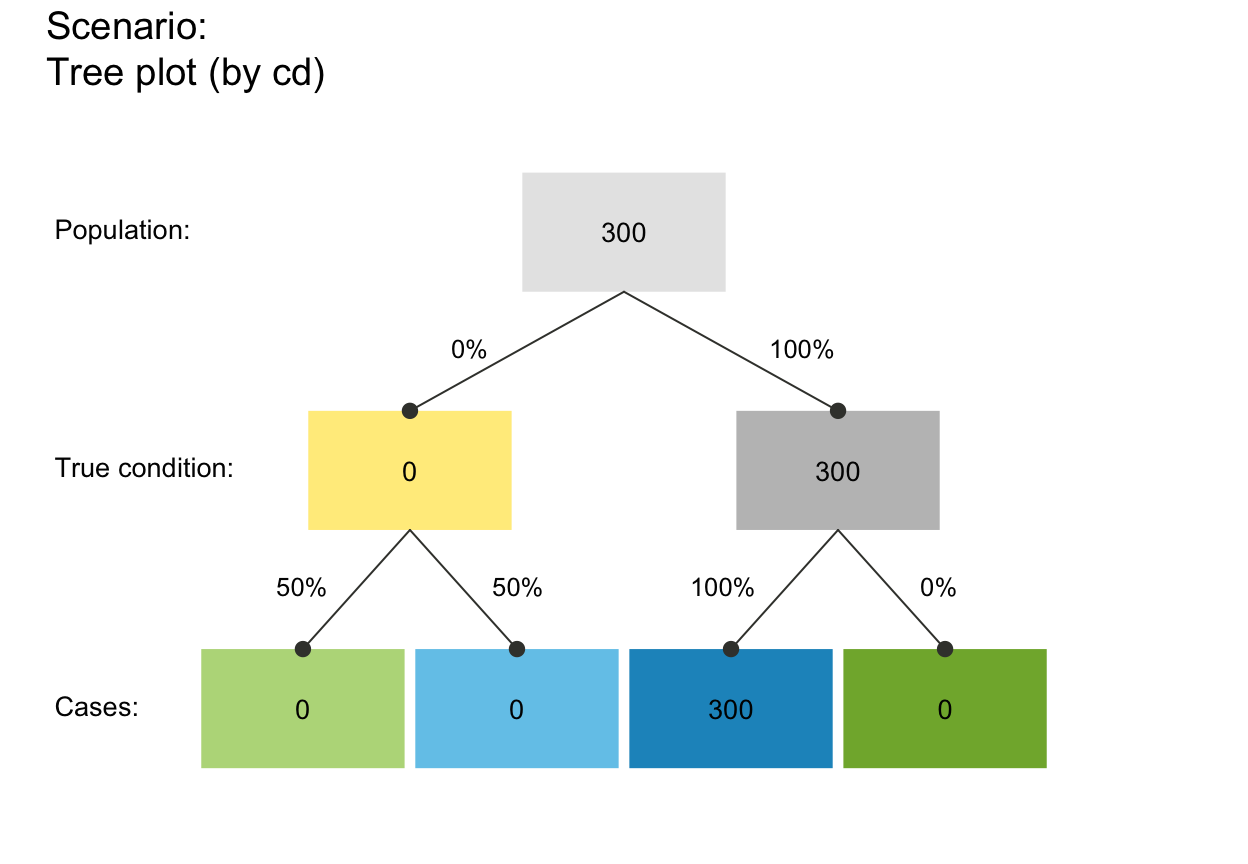# Variant: is_extreme_prob_set(0, sens, NA, NA, 1) # => TRUE + warning: N false positives#> Warning: Extreme case (prev = 0 & spec = 0): #> N fa (FP) cases; 0 cond_true or dec_true cases; PPV = NaN.#>  TRUEplot_tree(0, sens, NA, NA, 1, N = 350) # => illustrates this case#> Function 'plot_tree' is deprecated; using 'plot_prism' (with by = 'cd'/'dc'/'ac') instead.#> Warning: Extreme case (prev = 0 & spec = 0): #> N fa (FP) cases; 0 cond_true or dec_true cases; PPV = NaN.#> Warning: Extreme case (prev = 0 & spec = 0): #> N fa (FP) cases; 0 cond_true or dec_true cases; PPV = NaN.#> Warning: NPV is NaN.#> Warning: FOR is NaN.#> Warning: Some derived prob values are peculiar. Check for extreme probabilities!#> Warning: accu$mcc: A denominator of 0 was corrected to 1, resulting in mcc = 0.#> Warning: Argument (prob) is no probability.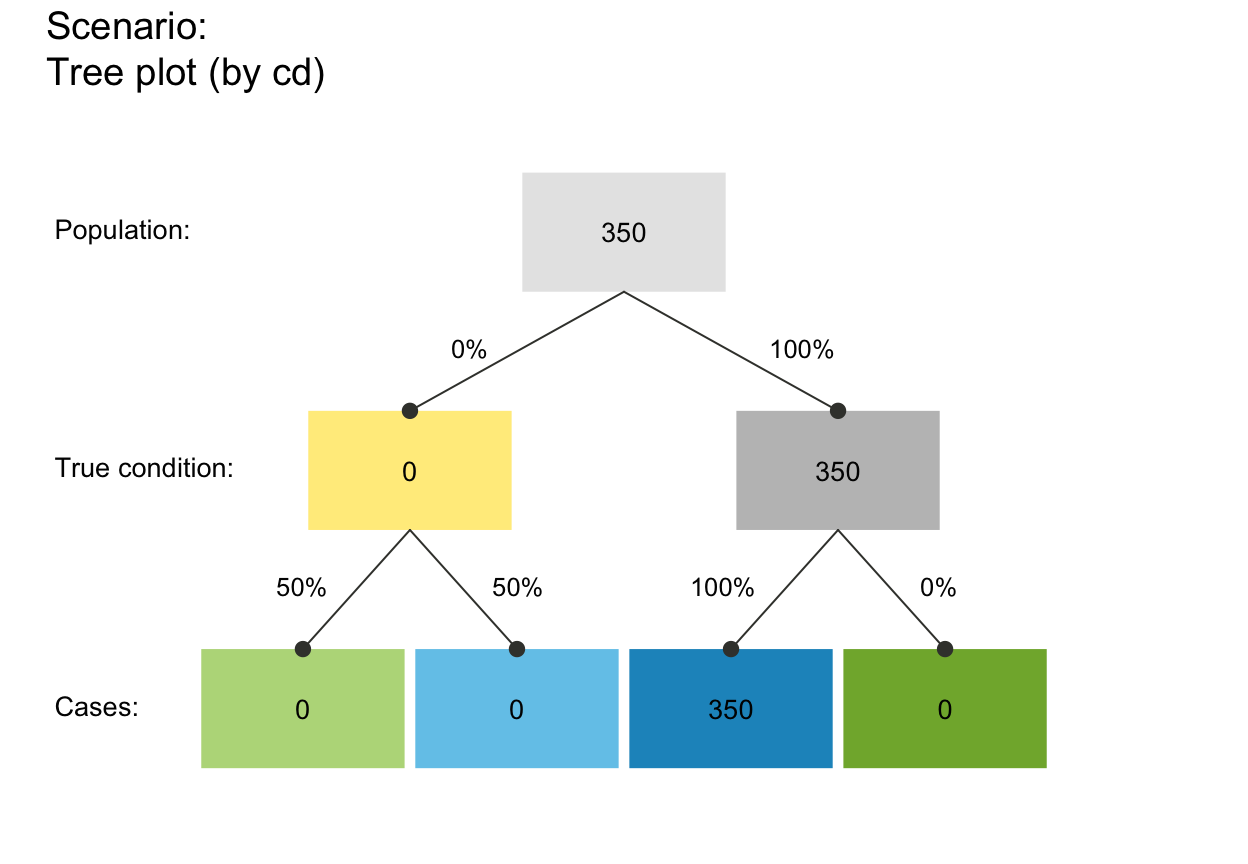sens <- .50
is_extreme_prob_set(0, sens, NA, 1)        # => TRUE + warning: N true negatives#> Warning: Extreme case (prev = 0 & spec = 1):
#>   N cr (TN) cases; 0 cond_true or dec_false cases; NPV = NaN.#>  TRUEplot_tree(0, sens, NA, NA, 1, N = 400)     # => illustrates this case#> Function 'plot_tree' is deprecated; using 'plot_prism' (with by = 'cd'/'dc'/'ac') instead.#> Warning: Extreme case (prev = 0 & spec = 0):
#>   N fa (FP) cases; 0 cond_true or dec_true cases; PPV = NaN.#> Warning: Extreme case (prev = 0 & spec = 0):
#>   N fa (FP) cases; 0 cond_true or dec_true cases; PPV = NaN.#> Warning: NPV is NaN.#> Warning: FOR is NaN.#> Warning: Some derived prob values are peculiar. Check for extreme probabilities!#> Warning: accu$mcc: A denominator of 0 was corrected to 1, resulting in mcc = 0.#> Warning: Argument (prob) is no probability.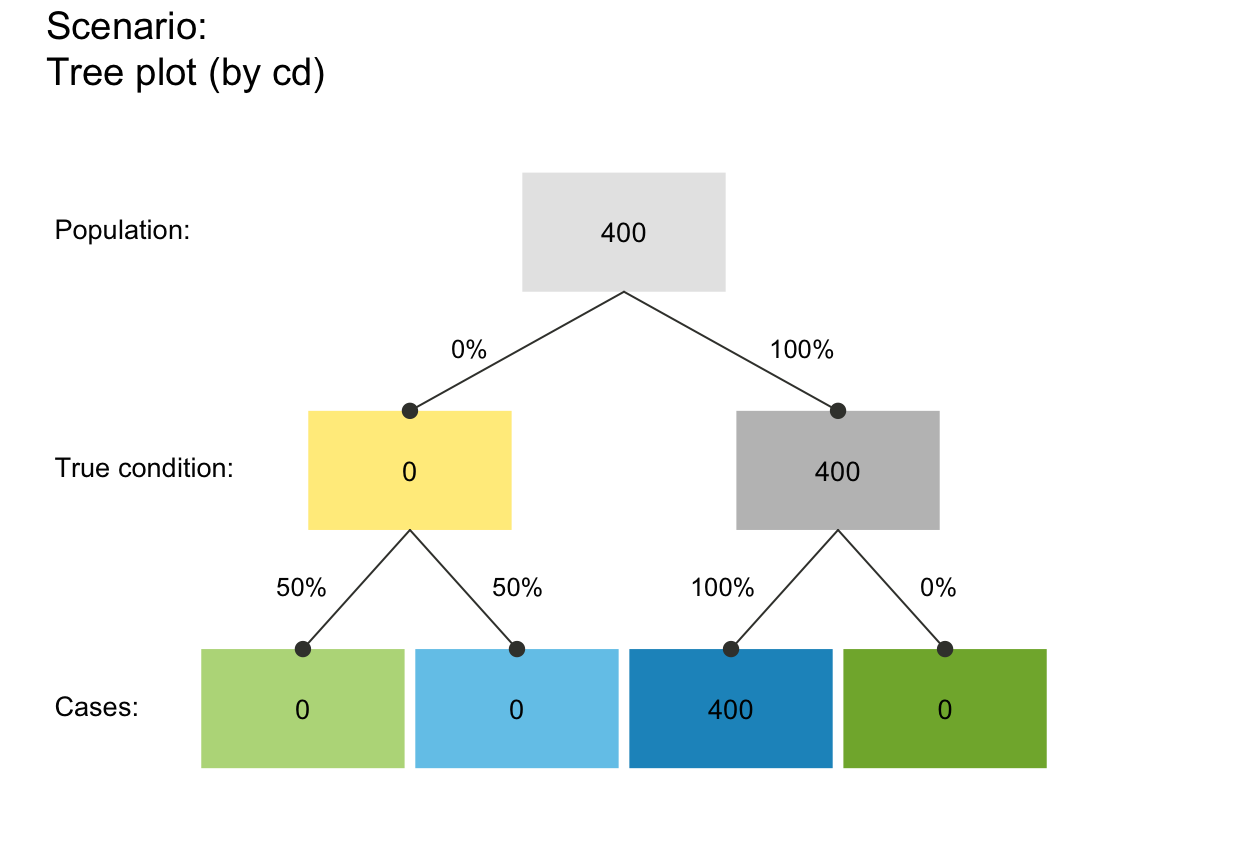# Variant: is_extreme_prob_set(0, sens, NA, NA, 0) # => TRUE + warning: N true negatives#> Warning: Extreme case (prev = 0 & spec = 1): #> N cr (TN) cases; 0 cond_true or dec_false cases; NPV = NaN.#>  TRUEplot_tree(0, sens, NA, NA, 0, N = 450) # => illustrates this case#> Function 'plot_tree' is deprecated; using 'plot_prism' (with by = 'cd'/'dc'/'ac') instead.#> Warning: Extreme case (prev = 0 & spec = 1): #> N cr (TN) cases; 0 cond_true or dec_false cases; NPV = NaN.#> Warning: Extreme case (prev = 0 & spec = 1): #> N cr (TN) cases; 0 cond_true or dec_false cases; NPV = NaN.#> Warning: PPV is NaN.#> Warning: FDR is NaN.#> Warning: Some derived prob values are peculiar. Check for extreme probabilities!#> Warning: accu$mcc: A denominator of 0 was corrected to 1, resulting in mcc = 0.#> Warning: Argument (prob) is no probability.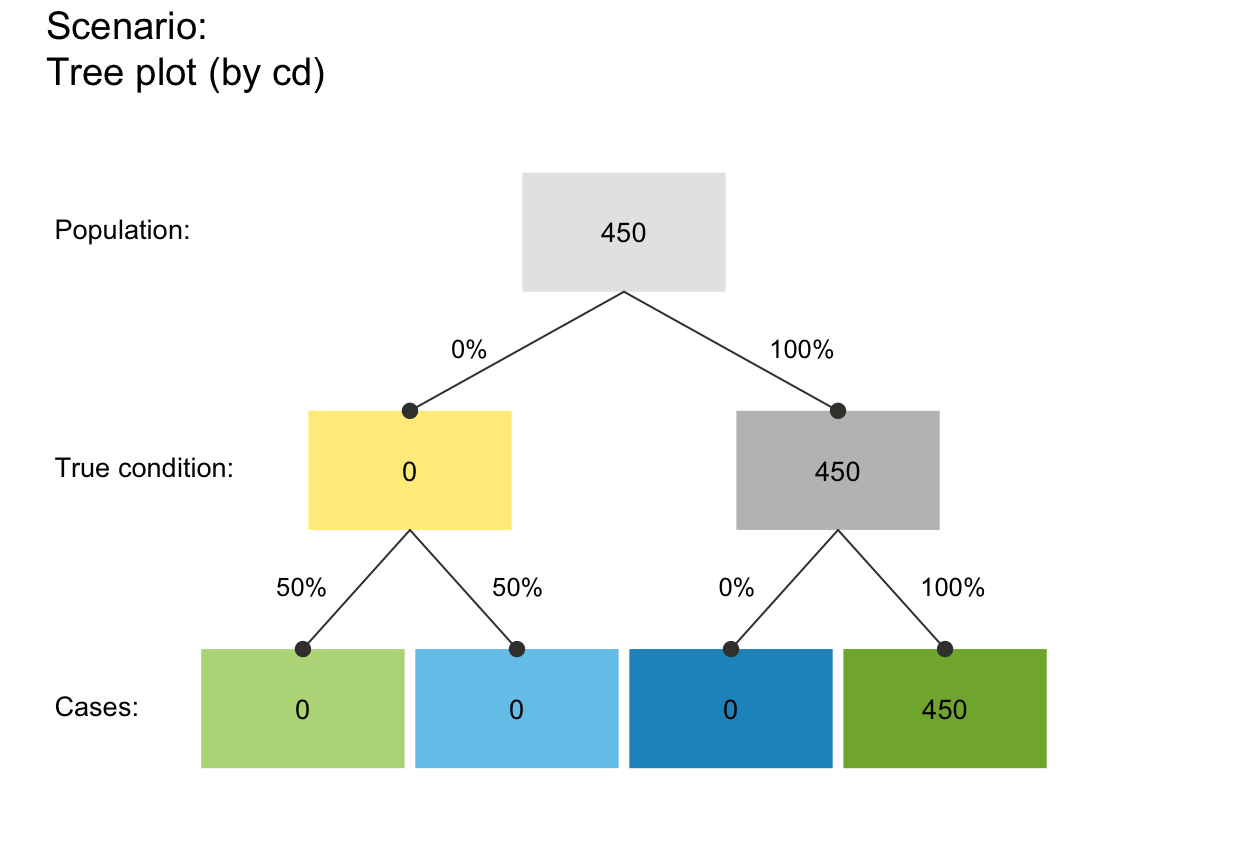prev <- .50
is_extreme_prob_set(prev, 0, NA, 1, NA)    # => TRUE + warning: 0 hi and 0 fa (0 dec_pos cases)#> Warning: Extreme case (sens = 0 & spec = 1):
#>   0 hi (TP) and 0 fa (FP) cases; 0 dec_pos cases; PPV = NaN.#>  TRUEplot_tree(prev, 0, NA, 1, NA, N = 500)     # => illustrates this case#> Function 'plot_tree' is deprecated; using 'plot_prism' (with by = 'cd'/'dc'/'ac') instead.#> Warning: Extreme case (sens = 0 & spec = 1):
#>   0 hi (TP) and 0 fa (FP) cases; 0 dec_pos cases; PPV = NaN.#> Warning: Extreme case (sens = 0 & spec = 1):
#>   0 hi (TP) and 0 fa (FP) cases; 0 dec_pos cases; PPV = NaN.#> Warning: PPV is NaN.#> Warning: FDR is NaN.#> Warning: Some derived prob values are peculiar. Check for extreme probabilities!#> Warning: accu$mcc: A denominator of 0 was corrected to 1, resulting in mcc = 0.#> Warning: Argument (prob) is no probability.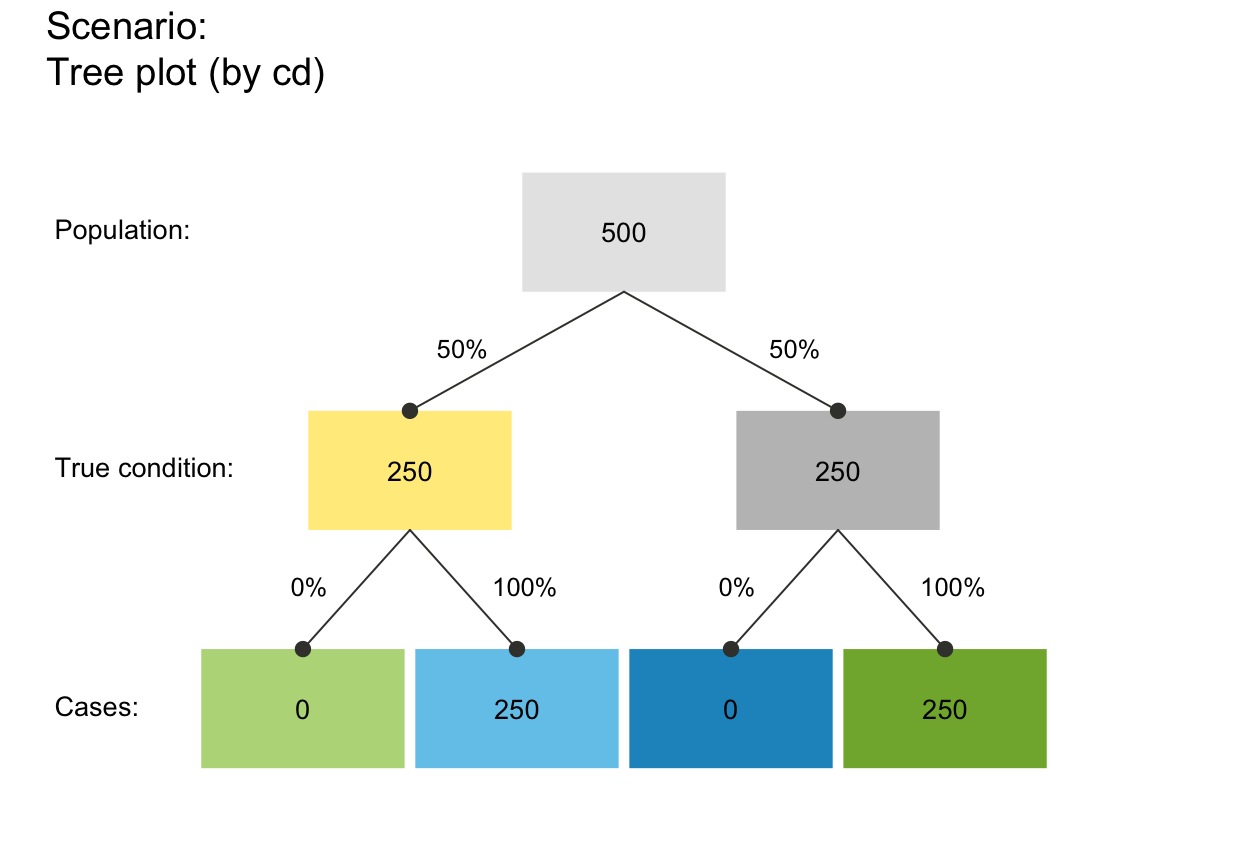# # Variant: is_extreme_prob_set(prev, 0, 0, NA, 0) # => TRUE + warning: 0 hi and 0 fa (0 dec_pos cases)#> Warning: Extreme case (sens = 0 & spec = 1): #> 0 hi (TP) and 0 fa (FP) cases; 0 dec_pos cases; PPV = NaN.#>  TRUEplot_tree(prev, 0, NA, 1, NA, N = 550) # => illustrates this case#> Function 'plot_tree' is deprecated; using 'plot_prism' (with by = 'cd'/'dc'/'ac') instead.#> Warning: Extreme case (sens = 0 & spec = 1): #> 0 hi (TP) and 0 fa (FP) cases; 0 dec_pos cases; PPV = NaN.#> Warning: Extreme case (sens = 0 & spec = 1): #> 0 hi (TP) and 0 fa (FP) cases; 0 dec_pos cases; PPV = NaN.#> Warning: PPV is NaN.#> Warning: FDR is NaN.#> Warning: Some derived prob values are peculiar. Check for extreme probabilities!#> Warning: accu$mcc: A denominator of 0 was corrected to 1, resulting in mcc = 0.#> Warning: Argument (prob) is no probability.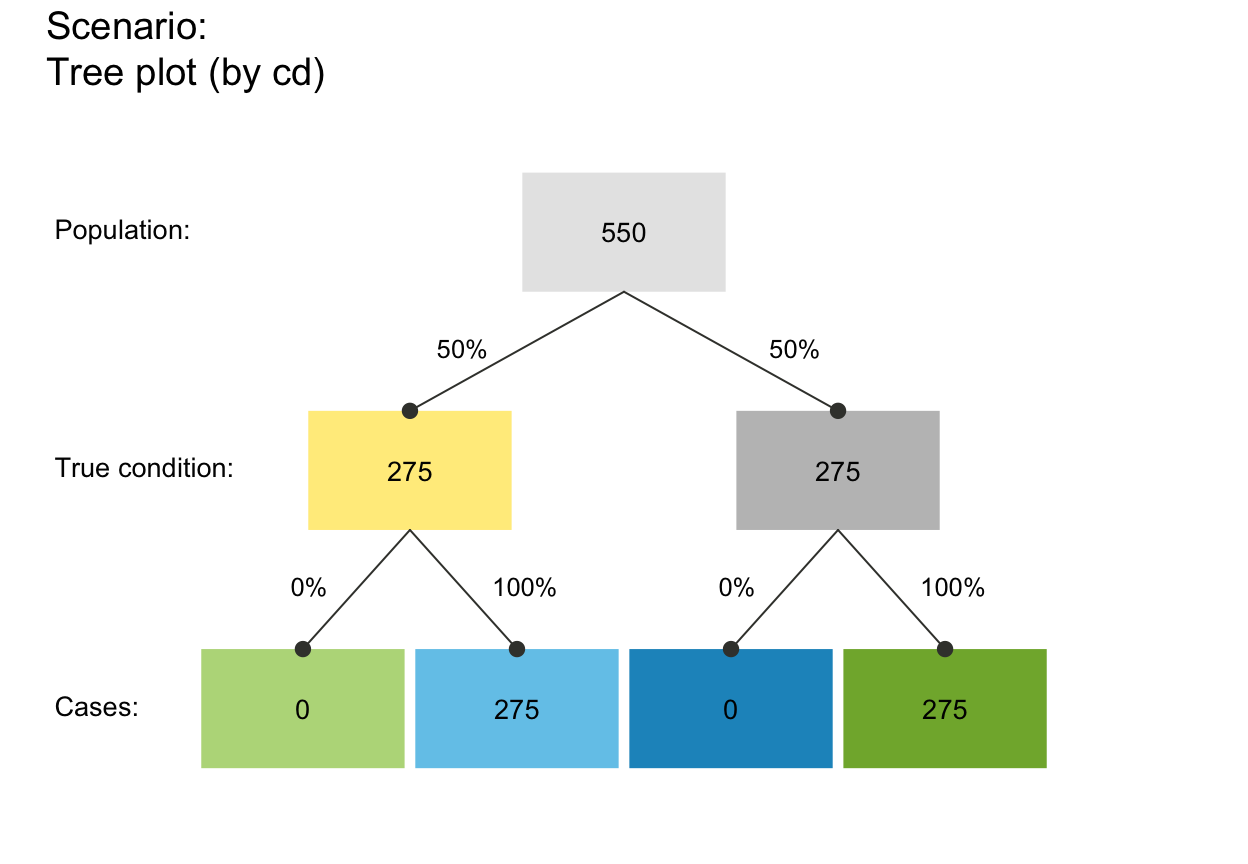prev <- .50
is_extreme_prob_set(prev, 1, NA, 0, NA)    # => TRUE + warning: 0 mi and 0 cr (0 dec_neg cases)#> Warning: Extreme case (sens = 1 & spec = 0):
#>   0 mi (FN) and 0 cr (TN) cases; 0 dec_neg cases; NPV = NaN.#>  TRUEplot_tree(prev, 1, NA, 0, NA, N = 600)     # => illustrates this case#> Function 'plot_tree' is deprecated; using 'plot_prism' (with by = 'cd'/'dc'/'ac') instead.#> Warning: Extreme case (sens = 1 & spec = 0):
#>   0 mi (FN) and 0 cr (TN) cases; 0 dec_neg cases; NPV = NaN.#> Warning: Extreme case (sens = 1 & spec = 0):
#>   0 mi (FN) and 0 cr (TN) cases; 0 dec_neg cases; NPV = NaN.#> Warning: NPV is NaN.#> Warning: FOR is NaN.#> Warning: Some derived prob values are peculiar. Check for extreme probabilities!#> Warning: accu$mcc: A denominator of 0 was corrected to 1, resulting in mcc = 0.#> Warning: Argument (prob) is no probability.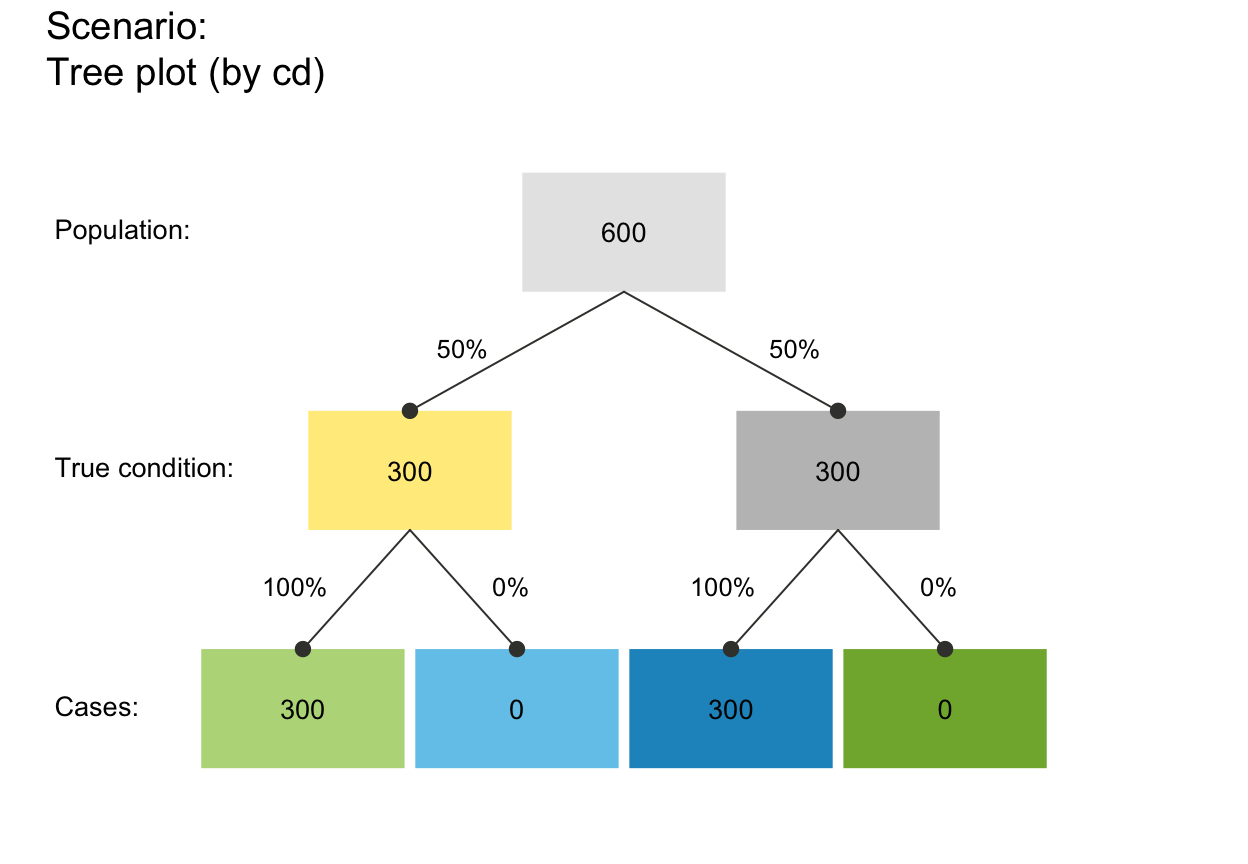# # Variant: is_extreme_prob_set(prev, 1, NA, 0, NA) # => TRUE + warning: 0 mi and 0 cr (0 dec_neg cases)#> Warning: Extreme case (sens = 1 & spec = 0): #> 0 mi (FN) and 0 cr (TN) cases; 0 dec_neg cases; NPV = NaN.#>  TRUEplot_tree(prev, 1, NA, 0, NA, N = 650) # => illustrates this case#> Function 'plot_tree' is deprecated; using 'plot_prism' (with by = 'cd'/'dc'/'ac') instead.#> Warning: Extreme case (sens = 1 & spec = 0): #> 0 mi (FN) and 0 cr (TN) cases; 0 dec_neg cases; NPV = NaN.#> Warning: Extreme case (sens = 1 & spec = 0): #> 0 mi (FN) and 0 cr (TN) cases; 0 dec_neg cases; NPV = NaN.#> Warning: NPV is NaN.#> Warning: FOR is NaN.#> Warning: Some derived prob values are peculiar. Check for extreme probabilities!#> Warning: accu$mcc: A denominator of 0 was corrected to 1, resulting in mcc = 0.#> Warning: Argument (prob) is no probability.